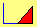SPREAD POSITIONS

The purpose of this lesson is to follow along and recreate the strategies developed here.

The third type of strategy is called a spread position.  A spread is  a portfolio of calls (a call spread) or a portfolio of puts (a put spread).  In a call spread, you buy and sell different call options.  If they differ only by the strike price, this would be a vertical call spread.  The term vertical comes from the way option prices are sometimes reported.  The different strike prices are listed vertically, and the maturities are listed horizontally,  as in the following Table.

Option Price Reporting and Vertical/Horizontal Spreads

 Expiration Date 1 Expiration Date 2 Strike 1 (K1) Option Price Option Price Strike 2 ( K2 ) Option Price Option Price

Following this terminology, a horizontal (or calendar) spread would fix the strike price (K) but vary the expiration date.  A diagonal  spread  would vary both strike price and expiration date.

Spreads come in two other varieties: bullish and bearish.  As the  names imply, if you (mainly) make  money  when  the  price  of  the  underlying security rises, you have a bullish position; and if you mainly make money when the price falls, you have a bearish position.  These positions are shown in following Figures 1-4.

Figure 1

Bullish Call Spread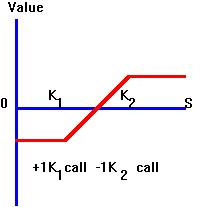Figure 2

Bearish Call Spread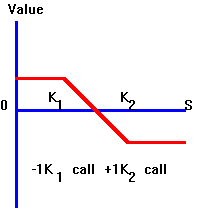Figure 3

Bullish Put Spread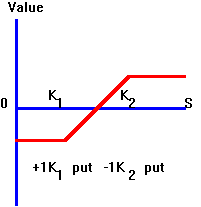Figure 4

Bearish Put Spread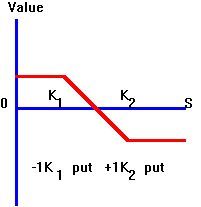Other types of spread positions are ratio spreads, where the ratio is the number of calls (puts) bought at a higher strike per call (put) sold at a lower strike.  The writer of such a portfolio has a ratio back spread.  Figures 5 and 6 show the payoffs from a -1:2 call ratio spread and a 2:-1 put ratio spread.  Observe that in these positions a floor is imposed upon possible losses, whereas the position participates in any upside gain.  In the -1:2 call ratio spread depicted in Figure 8.10, no upper bound is imposed on the upside potential.

Figure 5

-1:2 Call Ratio Spread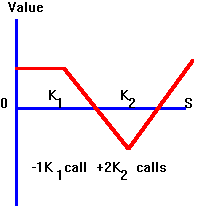The 2:-1 put spread in Figure 6 imposes an upper bound on the upside gain.

Figure 6

2:-1 Put Ratio Spread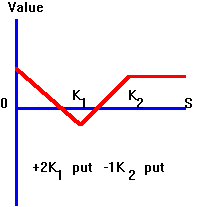Spreads can also be used to make profits when prices are expected to remain in a stable range.  A famous example of this is the butterfly spread, which consists of buying a low and high strike-price call and selling two medium strike-price calls.  The payoff from this position is depicted in the following Figure 7.

Figure 7

Call Butterfly Spread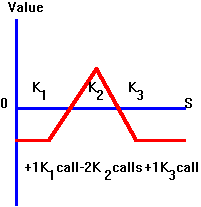The position in Figure 7 can also be engineered by selling the medium puts and buying the extreme strike-price puts, as shown in the following Figure 8.

Figure 8

Put Butterfly Spread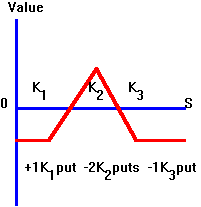Calendar, or horizontal, spreads are difficult to illustrate, as  the expiration dates of the options are  different.  However, you can view the payoffs from such strategies in Option Tutor.   An option pricing model such as the Black-Scholes lets you value the long-dated option at the expiration date of the short-dated option, and examine the resulting payoffs as a function of the underlying stock price.

OS Financial Trading System, PO Box 11356 , Pittsburgh , PA 15238 USA , Phone 1-800-967-9897, Fax 1-412-967-5958, Email fts@ftsweb.com,

Restart to return home

©2003 OS Financial Trading System